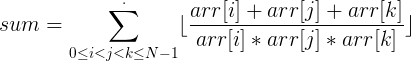Velma and Triplets
Tag(s):

## Ad-Hoc, Combinatorics, Combinatorics basics, Easy, Implementation, Mathematics

Problem
Editorial
Analytics

Given an array of size N, Velma give Scooby and Shaggy to compute the following sum on the array (0-indexed array).,
where $\lfloor.\rfloor$ is the floor operator.
Since it was taking too long for Scooby and Shaggy to compute the sum, they need your help.
Can you help them?

### Input

The first line of input will contain a single integer N, denoting the size of the array.
The second line will contain N integers arr0, arr1, arr2, ..., arrn-1.

### Output

Print a single integer, the answer to Velma's question.

### Constraints

• $1 \leq N \leq 100, 1 \leq arr_{i} \leq 1000$ in 40% of test cases.
• $1 \leq N \leq 3 \cdot 10^5, 1 \leq arr_{i} \leq 5*10^4$ in 60% of test cases.

Note: The answer will fit in signed 64-bit integer

SAMPLE INPUT
5
1 2 1 7 3
SAMPLE OUTPUT
6
Explanation

The following triplets exists in the given array:
1 2 1
1 2 7
1 2 3
1 1 7
1 1 3
1 7 3
2 1 7
2 1 3
2 7 3
1 7 3
So, if we compute the required sum using these triplets, we get the sum to be equal to 6.

Time Limit: 1.0 sec(s) for each input file.
Memory Limit: 256 MB
Source Limit: 1024 KB
Marking Scheme: Marks are awarded when all the testcases pass.
Allowed Languages: Bash, C, C++, C++14, Clojure, C#, D, Erlang, F#, Go, Groovy, Haskell, Java, Java 8, JavaScript(Rhino), JavaScript(Node.js), Julia, Kotlin, Lisp, Lisp (SBCL), Lua, Objective-C, OCaml, Octave, Pascal, Perl, PHP, Python, Python 3, R(RScript), Racket, Ruby, Rust, Scala, Swift, Swift-4.1, TypeScript, Visual Basic

## CODE EDITOR

Initializing Code Editor...

## This Problem was Asked inChallenge Name

October Circuits

OTHER PROBLEMS OF THIS CHALLENGE
• Basic Programming > Implementation
• Algorithms > Graphs
• Algorithms > Graphs
• Algorithms > Graphs
• Algorithms > Graphs
• Math > Basic Math